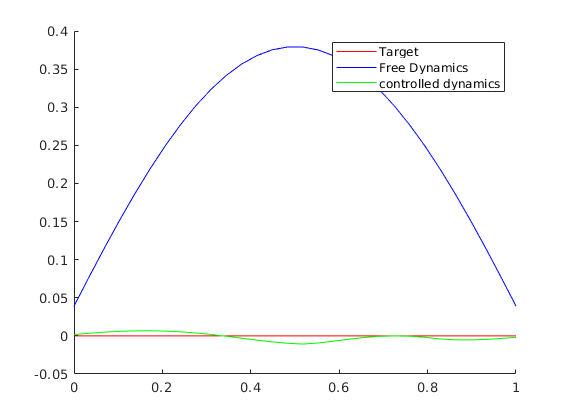# Control for a semilinear heat equation and analogies with a collective behavior model

To start this tutorial, write the following command in the MATLAB console

open T0007_semilinear

## Semi-linear semi-discrete heat equation and collective behavior

In this tutorial we will apply the DyCon toolbox to find a control to the semi-discrete semi-linear heat equation.

where $N^2A$ is the discretization of the Laplacian in 1d in $N$ nodes. We are looking for a control that after time $T$ steers the system near zero. In order to do so we will frame the problem as a minimization of a functional and we will apply gradient descent to find it. Note that the convexity of the fuctional is not proven, therefore, we will obtain a local minima for the functional. The functional considered will be:

where by $| \cdot |_{L^2}$ we understand the discrete $L^2$ norm.

Once this control is computed for a certain N, we will think on a dynamical system that models an opinion dynamics with $N$ agents communicating through a chain.

\begin{equation}\label{m1}y_t-\frac{1}{N}Ay=G(y)+Bv\end{equation}

The goal will be to compute also the control $v$ thinking model \eqref{m1} as if it was a semidiscretization of a heat equation with diffusivity $\frac{1}{N^3}$. Furthermore we will also compute the control for model \eqref{m1} with the non-linearity being non-homogeneous on $N$ and a time horizon being $T_N=N^3T$.

Definition of the time

syms t
%% Discretization of the space
N = 30;
xi = 0; xf = 1;
xline = linspace(xi,xf,N);


Interior Control region between 0.5 and 0.8

w1=0.5;
w2=0.8;


Here we count how many elements in the discretization should be placed inside the control region

count=1;
for i=1:N
if (double(i-1))/double(N) > w1
if (i-1)/double(N) < w2
count=count+1;
end
end
end


we define symbolically the vectors of the state and the control

symY = SymsVector('y',N);
symU = SymsVector('u',count);


We create the functional that we want to minimize Our goal is to set the system to zero penalizing the norm of the control by a parameter $\beta$ that will be small.

YT = 0*xline.';

dx = xline(2) - xline(1);
beta = dx^4;
%%
Psi  = @(T,Y)   (1/beta)*(YT - Y).'*(YT - Y);
L    = @(t,Y,U) (U.'*U)*(abs(w1-w2))/count;


We create the ODE object Our ODE object will have the semi-discretization of the semilinear heat equation. We set also initial conditions, define the non linearity and the interaction of the control to the dynamics.

Initial condition

Y0 = 2*sin(pi*xline');


Diffusion part: the discretization of the 1d Laplacian

A=(N^2)*(full(gallery('tridiag',N,1,-2,1)));


We define the matrix B that will be the effect of the interior control to the dynamics

B = zeros(N,count);
count2=1;
for i=1:N
if (i-1)/double(N) >= w1
if (i-1)/double(N) < w2
B(i,count2)=1;
count2=count2+1;
end
end
end


Definition of the non-linearity $\partial_y[-5\exp(-y^2)]$

syms x;
syms G(x);
syms U(x);
syms DG(x);
U(x)=-5*exp(-x^2);
G(x)=diff(U,x);
formula=G(x);
G = symfun(formula,x)


G(x) =

10*x*exp(-x^2)



and we define the part of the dynamics corresponding to the nonlinearity

vectorF = arrayfun( @(x)G(x),symY);


Putting all the things together

Fsym  = A*symY + vectorF + B*symU;
F     = matlabFunction(Fsym,'Vars',{t,symY,symU,sym.empty});


Creation of the ODE object Time horizon

T = 1;


We create the ODE-object and we change the resolution to $dt=0.01$ in order to see the variation in a small time scale. We will get the values of the solution in steps of size odeEqn.dt, if we do not care about modifying this parameter in the object, we might get the solution in certain time steps that will hide part of the dynamics.

odeEqn = pde(F,symY,symU,'InitialCondition',Y0,'FinalTime',T);
odeEqn.mesh = xline;
odeEqn.Nt=30;
odeEqn.Solver = @ode23tb;


We solve the equation and we plot the free solution applying solve to odeEqn and we plot the free solution.

solve(odeEqn);

figure;
SIZ=size(odeEqn.StateVector.Numeric);
time=linspace(0,T,SIZ(1));
space=linspace(1,N,N);
[TIME,SPACE]=meshgrid(time,space);
surf(TIME',SPACE',odeEqn.StateVector.Numeric,'EdgeColor','none');
title('Free Dynamics')
ylabel('space discretization')
xlabel('Time')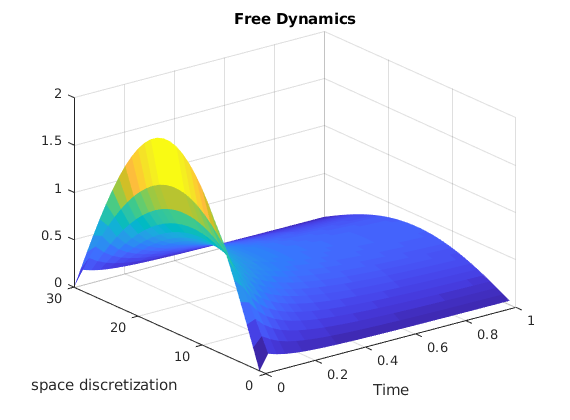We create the object that collects the formulation of an optimal control problem by means of the object that describes the dynamics odeEqn, the functional to minimize Jfun and the time horizon T

iCP1 = Pontryagin(odeEqn,Psi,L);


We apply the steepest descent method to obtain a local minimum (our functional might not be convex).

U0 = zeros(length(iCP1.Dynamics.tspan),iCP1.Dynamics.ControlDimension);
fminunc(@(U) Control2Functional(iCP1,U),U0,options);

                                                        First-order
Iteration  Func-count       f(x)        Step-size       optimality
0           1      1.59301e+06                      5.18e+05
1           2           911870    1.92945e-06       3.88e+05
2           5          12468.3           0.25       3.34e+04
3           7          6697.37            0.5       3.43e+04
4          11          5536.69         1.6639       3.33e+04
5          14          4537.42      0.0974533       3.06e+04
6          16          1484.09       0.396227        1.7e+04
7          18          1074.29       0.311396       1.63e+04
8          20          640.583       0.374707        1.3e+04
9          21          445.574              1       1.25e+04
10          23          398.673        0.34088       1.15e+04
11          25          368.836       0.289496       1.06e+04
12          54          361.771      0.0318763       1.03e+04

Local minimum possible.

fminunc stopped because it cannot decrease the objective function
along the current search direction.


figure;
SIZ=size(iCP1.Dynamics.StateVector.Numeric);
time=linspace(0,T,SIZ(1));
space=linspace(1,N,N);
[TIME,SPACE]=meshgrid(time,space);
surf(TIME',SPACE',iCP1.Dynamics.StateVector.Numeric,'EdgeColor','none')
title('Controlled Dynamics')
ylabel('space discretization')
xlabel('Time')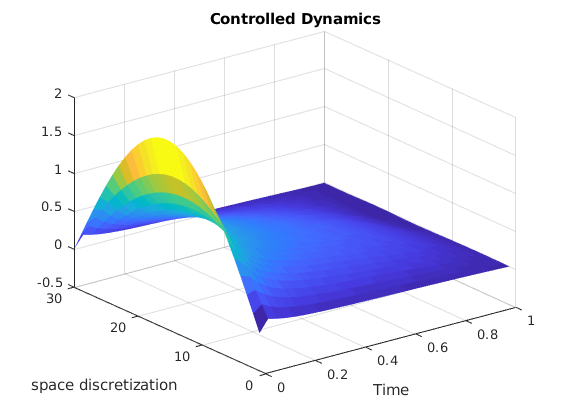The control function inside the control region

figure;
SIZ=size(iCP1.Dynamics.Control.Numeric);
time=linspace(0,T,SIZ(1));
space=linspace(1,SIZ(2)-1,SIZ(2)-1);
[TIME,SPACE]=meshgrid(time,space);
surf(TIME',SPACE',iCP1.Dynamics.Control.Numeric(:,1:SIZ(2)-1),'EdgeColor','none')
title('Control')
ylabel('space discretization')
xlabel('Time')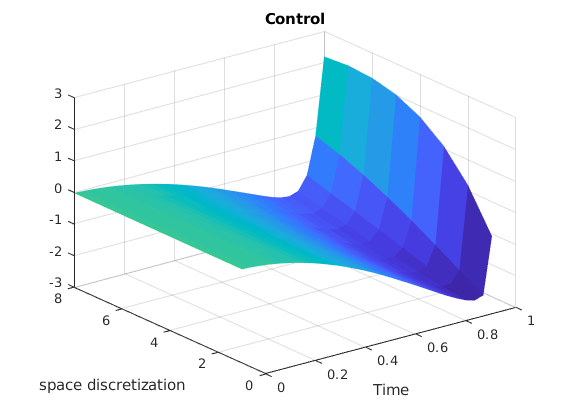figure;
line(xline,YT,'Color','red')
line(xline,odeEqn.StateVector.Numeric(end,:),'Color','blue')
line(xline,iCP1.Dynamics.StateVector.Numeric(end,:),'Color','green')
legend('Target','Free Dynamics','controlled dynamics')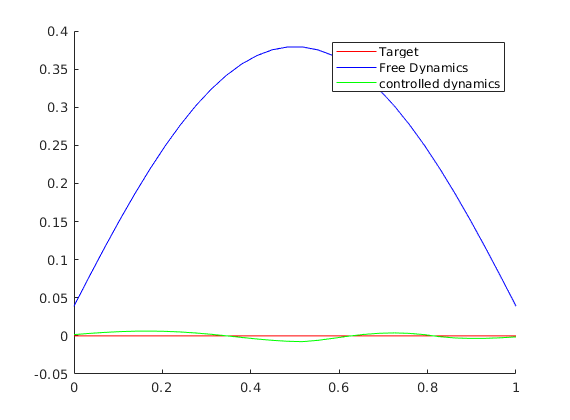Now we apply the same procedure for the collective behavior dynamics.

We will employ a function that does the algorithm explained before for the semilinear heat equation having the chance to set a diffusivity constant.

We set the parameters for the function

beta=0.0000001;
y0=@(x)2*sin(pi*x);
syms x
syms G(x);
syms U(x);
syms DG(x);
U(x)=-5*exp(-x^2);
G(x)=diff(U,x);
T=1;
N=30;


For the simulation of the model in collective behavior we will employ a diffusivity $D=\frac{1}{N^3}$.

[a,b,c,d]=SLSD1doptimalnullcontrol_T007_semilinear(N,1/(N^3),G,T,beta,[0.5,0.8],y0);

                                                        First-order
Iteration  Func-count       f(x)        Step-size       optimality
0           1          128.678                         0.149
1           3          111.576             10          0.111
2           4          99.9261              1         0.0807
3           5          89.1847              1         0.0379
4           7          87.0984       0.274087         0.0189
5           9          86.9148            0.5        0.00721
6          10          86.8984              1        0.00217
7          11          86.8968              1        0.00102
8          13          86.8955         3.8579        0.00113
9          14          86.8824              1        0.00763
10          16          86.8738       0.700036        0.00824
11          18          86.8605       0.644337         0.0122
12          19          86.8393              1        0.00537
13          21          86.8378            0.1        0.00829
14          22          86.8317              1        0.00828
15          23          86.8297              1        0.00272
16          25          86.8275            0.5        0.00429
17          27          86.8264       0.406898         0.0141
18          28          86.8238              1        0.00306
19          29          86.8212              1        0.00647
First-order
Iteration  Func-count       f(x)        Step-size       optimality
20          32            86.82       0.301714         0.0162
21          33          86.8156              1        0.00621
22          35          86.8143       0.491468         0.0197
23          37          86.8116       0.417456        0.00915
24          39          86.8098       0.672266         0.0111
25          41           86.805       0.481882         0.0247
26          43          86.8045       0.115993        0.00627
27          44          86.8016              1        0.00698
28          46          86.8014       0.433276         0.0415
29          48          86.8013            0.5         0.0543
30          51          86.7972       0.339269         0.0228
31          66          86.7952            0.1         0.0493

Local minimum possible.

fminunc stopped because it cannot decrease the objective function
along the current search direction.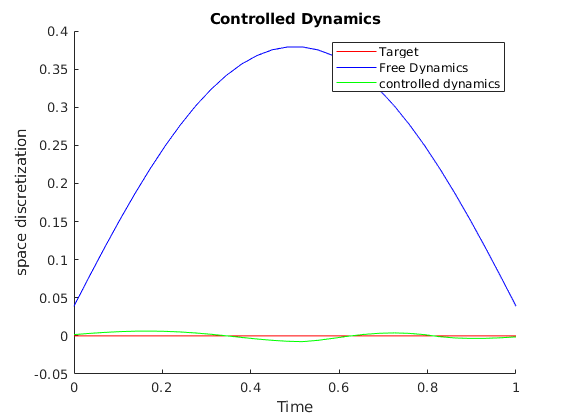figure;
surf(a.time,a.space,a.value,'EdgeColor','none');
title('Free Dynamics')
ylabel('space discretization')
xlabel('Time')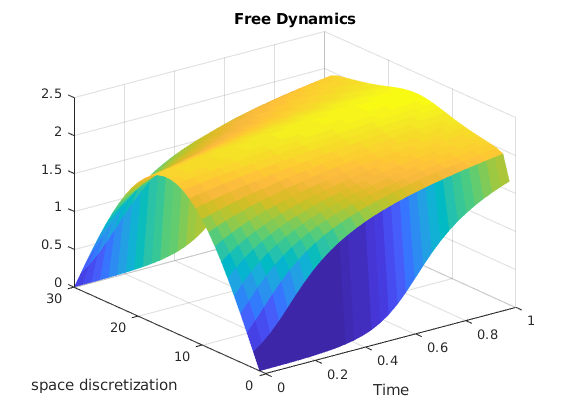figure;
surf(b.time,b.space,b.value,'EdgeColor','none')
title('Controlled Dynamics')
ylabel('space discretization')
xlabel('Time')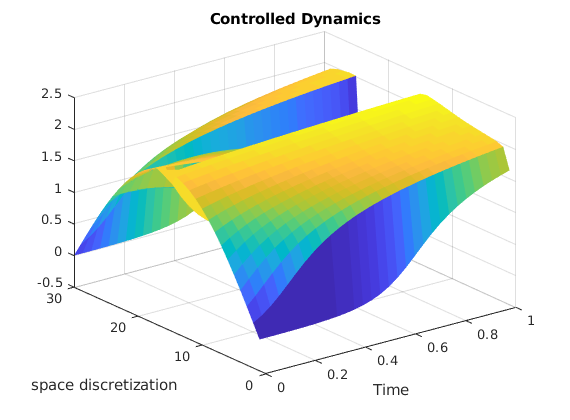figure;
surf(c.time,c.space,c.value,'EdgeColor','none')
title('Control')
ylabel('space discretization')
xlabel('Time')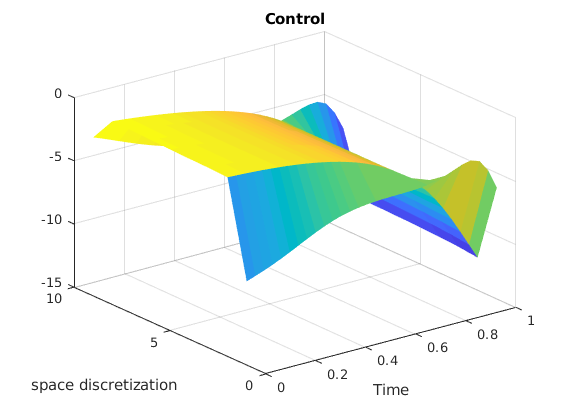xline = linspace(xi,xf,N);
figure;
line(xline,d.y1,'Color','red')
line(xline,d.y2,'Color','blue')
line(xline,d.y3,'Color','green')
legend('Target','Free Dynamics','controlled dynamics')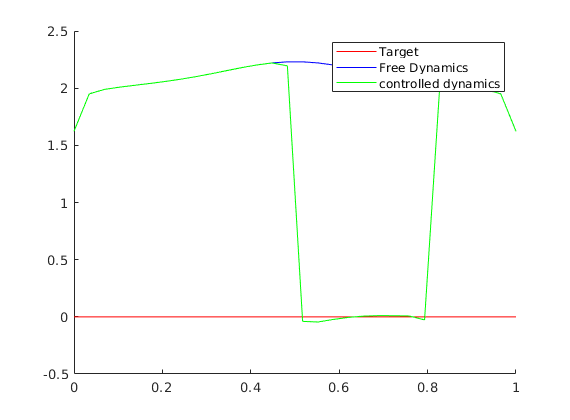Now we will change also the time horizon and we will incorporate a non-homogeneous non-linearity, we will just divide the non-linearity $G$ by $N^3$

[a,b,c,d]=SLSD1doptimalnullcontrol_T007_semilinear(N,1/(N^2),G/(N^2),N^2*T,beta,[0.5,0.8],y0);

                                                        First-order
Iteration  Func-count       f(x)        Step-size       optimality
0           1          2.25231                          22.7
1           5         0.270365    0.000118533           7.78
2           7        0.0182406       0.363002           1.62
3           8         0.015664              1           2.02
4           9        0.0147025              1           2.33
5          11        0.0132603       0.268426           2.21
6          12       0.00897682              1           1.95
7          13       0.00272977              1           1.11
8          14       0.00138325              1          0.709
9          15       0.00121058              1          0.341
10          16      0.000860606              1          0.439
11          17       0.00082607              1          0.641

Local minimum possible.

fminunc stopped because it cannot decrease the objective function
along the current search direction.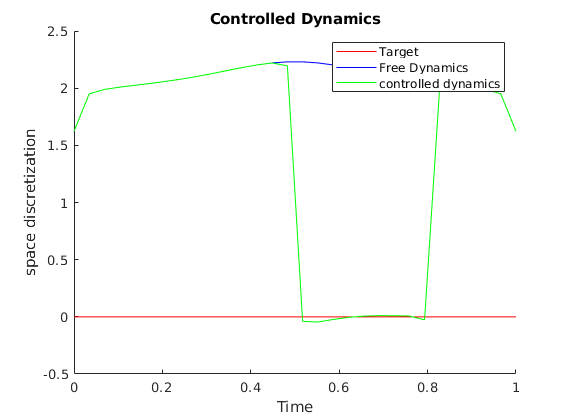figure;
surf(a.time,a.space,a.value,'EdgeColor','none');
title('Free Dynamics')
ylabel('space discretization')
xlabel('Time')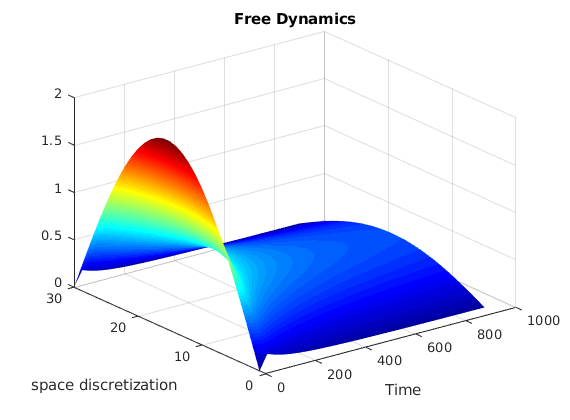figure;
surf(b.time,b.space,b.value,'EdgeColor','none')
title('Controlled Dynamics')
ylabel('space discretization')
xlabel('Time')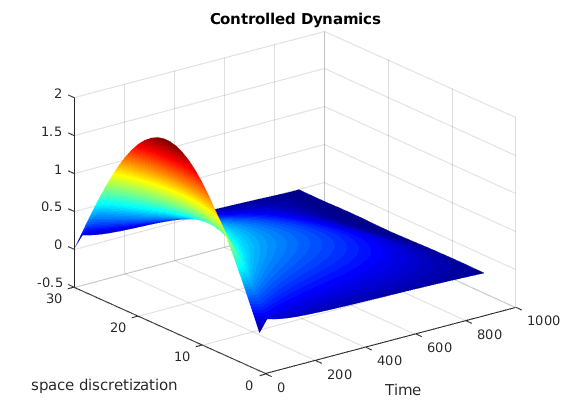figure;
surf(c.time,c.space,c.value,'EdgeColor','none')
title('Control')
ylabel('space discretization')
xlabel('Time')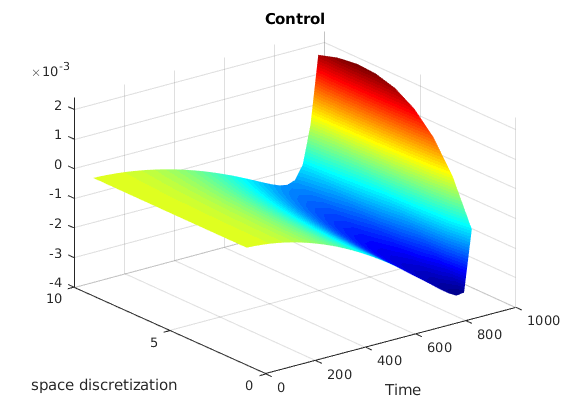figure;
xline = linspace(xi,xf,N);
line(xline,d.y1,'Color','red')
line(xline,d.y2,'Color','blue')
line(xline,d.y3,'Color','green')
legend('Target','Free Dynamics','controlled dynamics')